### 一、题目描述

输入：s = "We are happy."

• 0 <= s 的长度 <= 10000

### 四、图片描述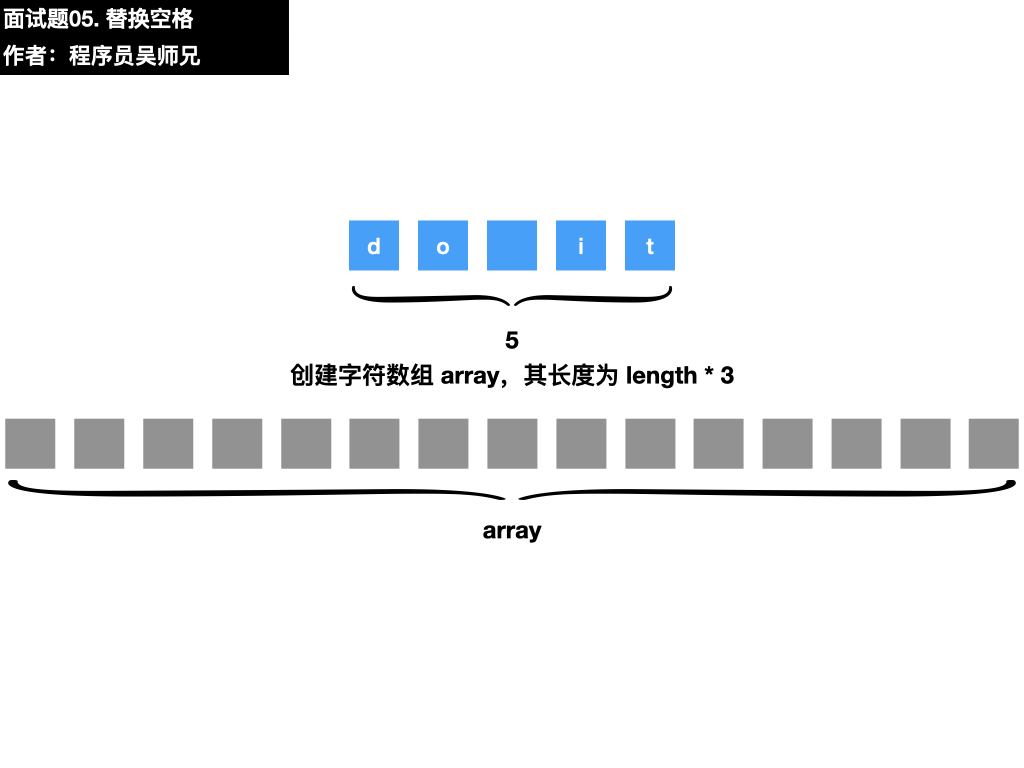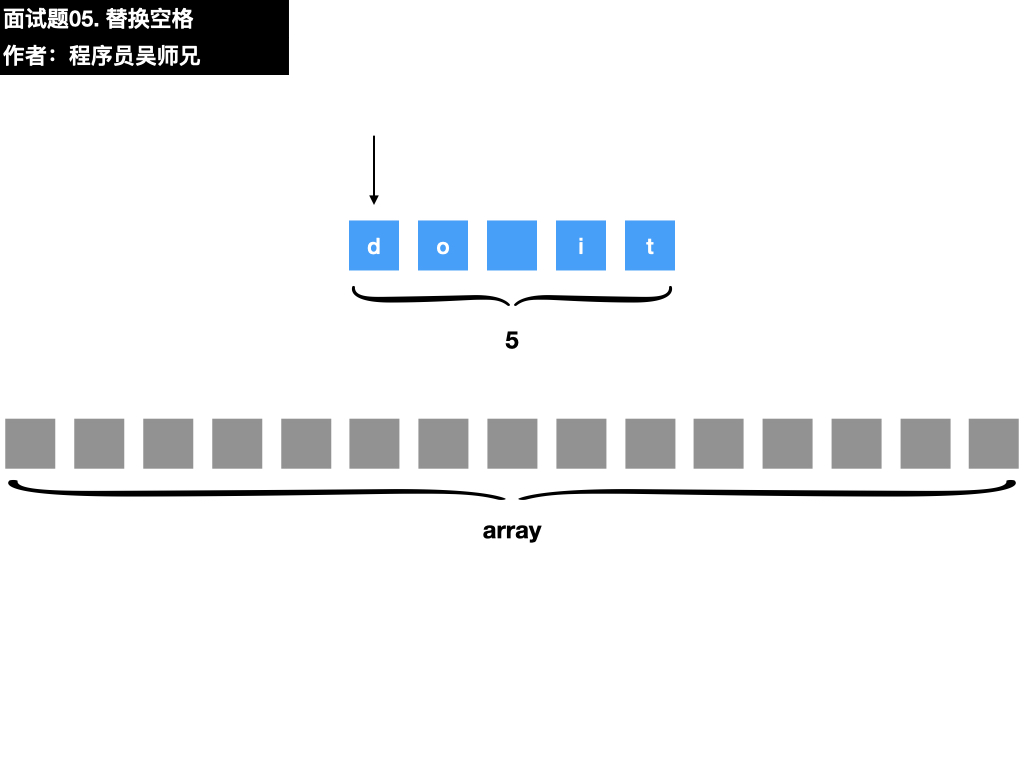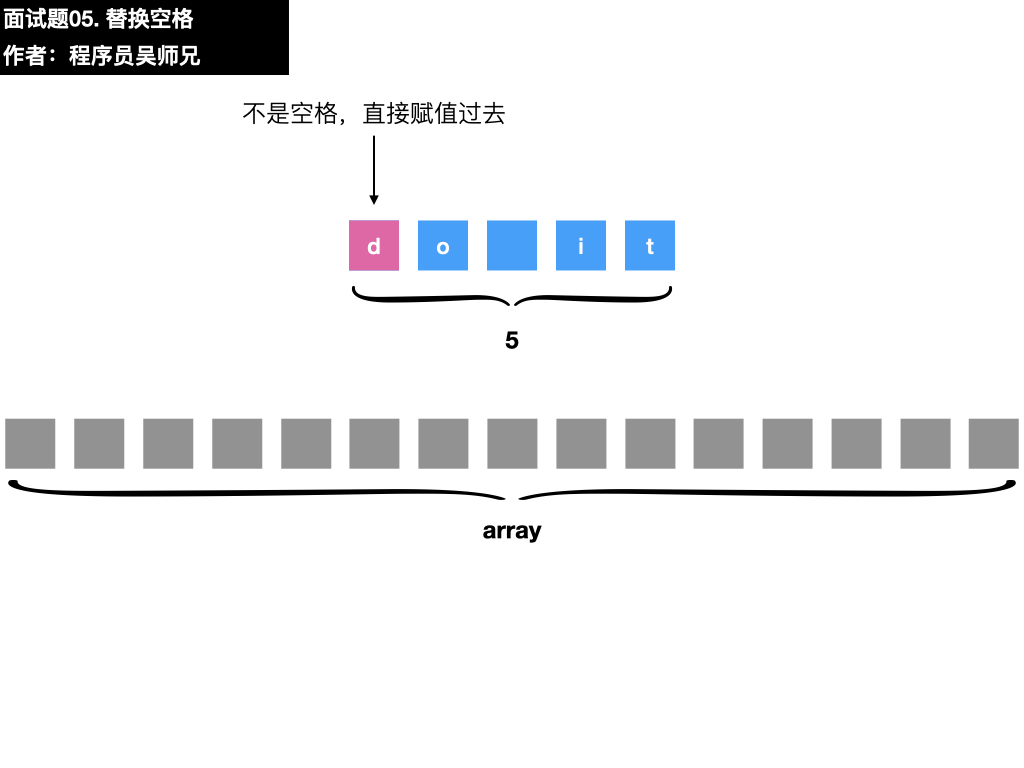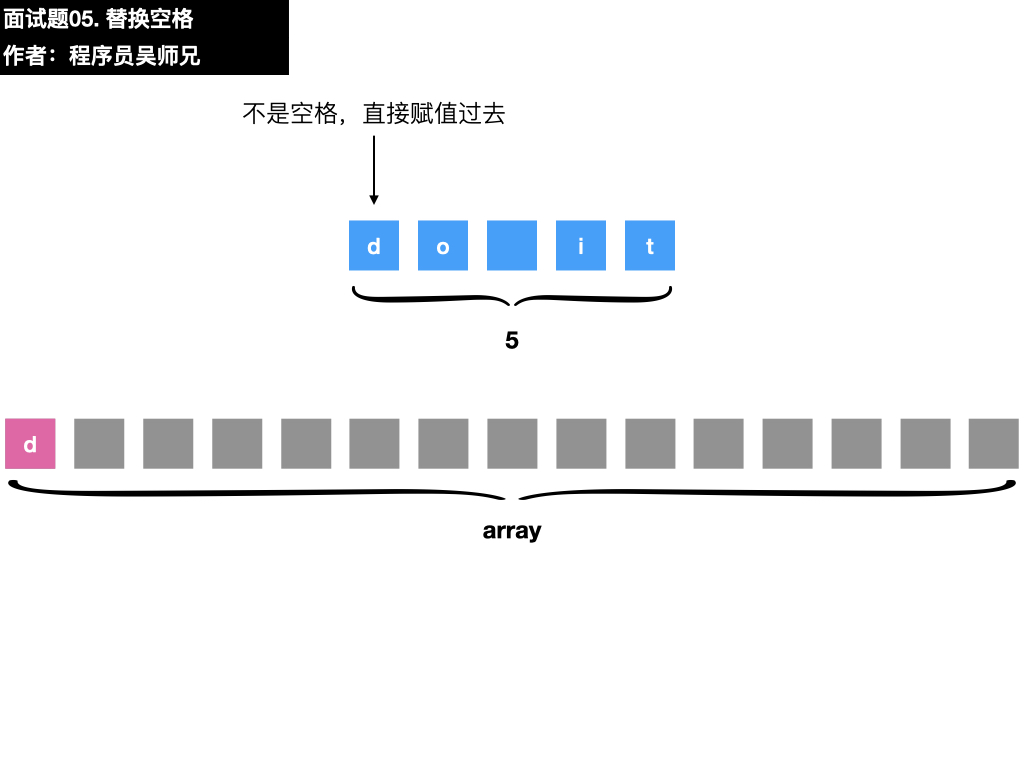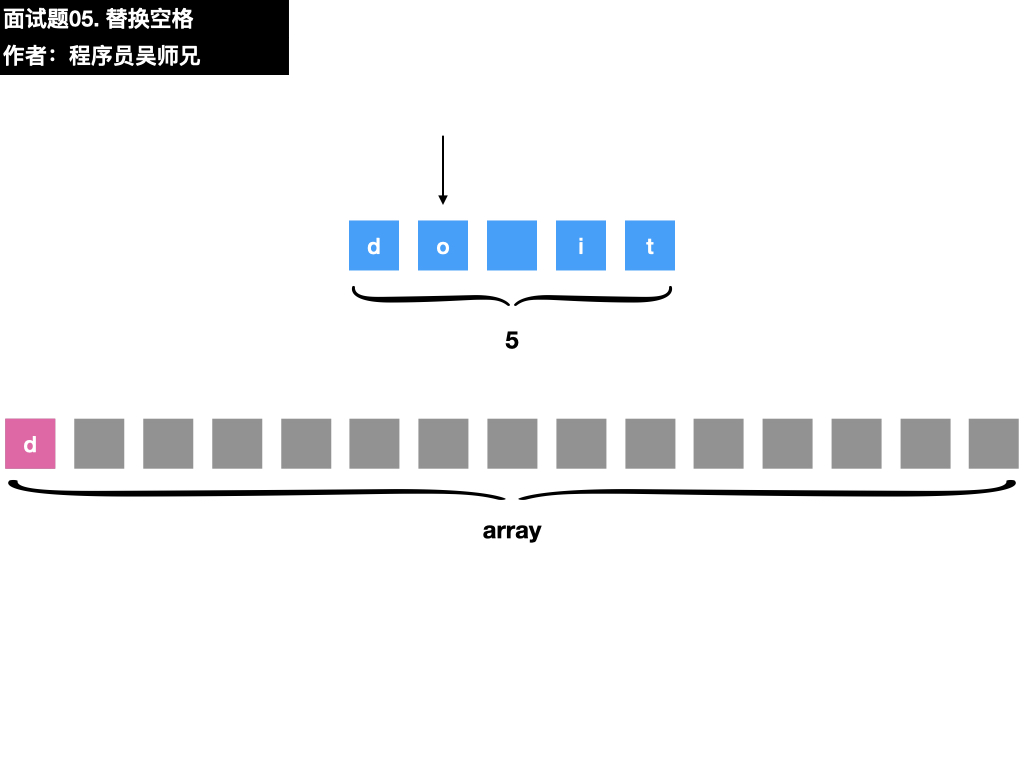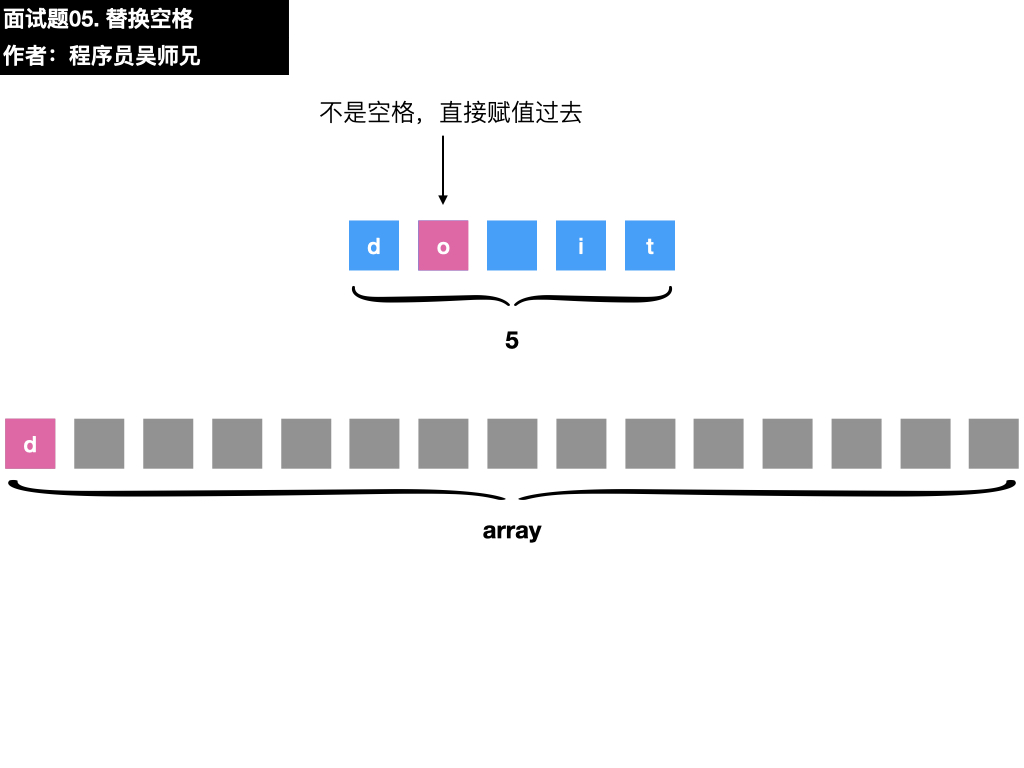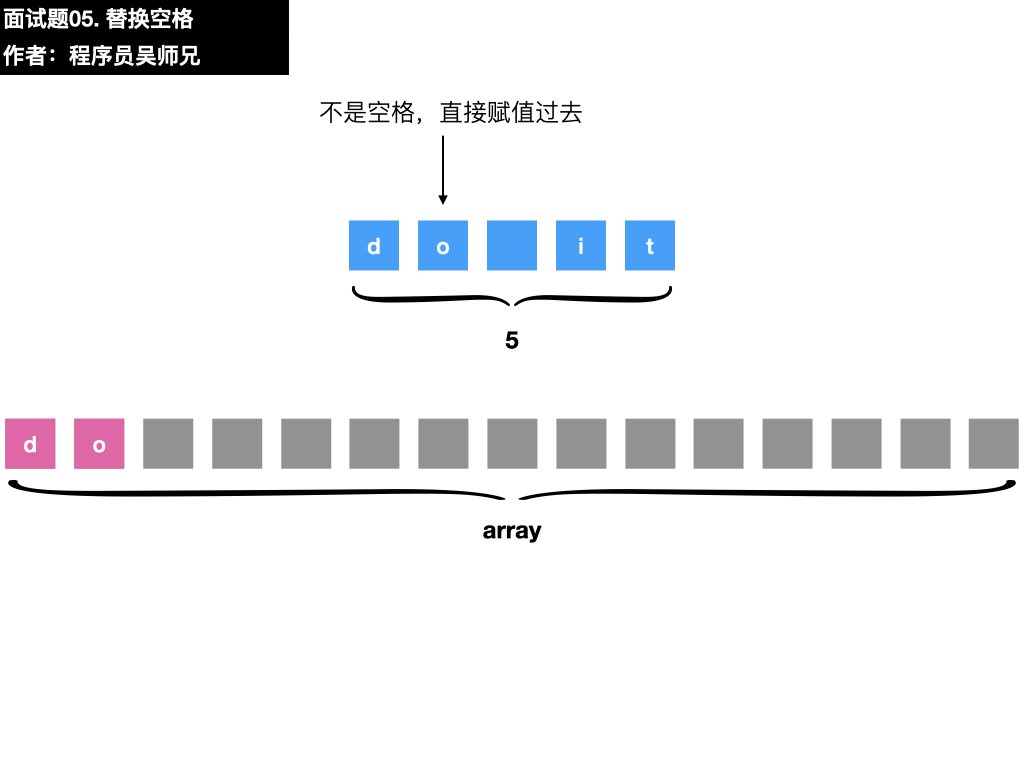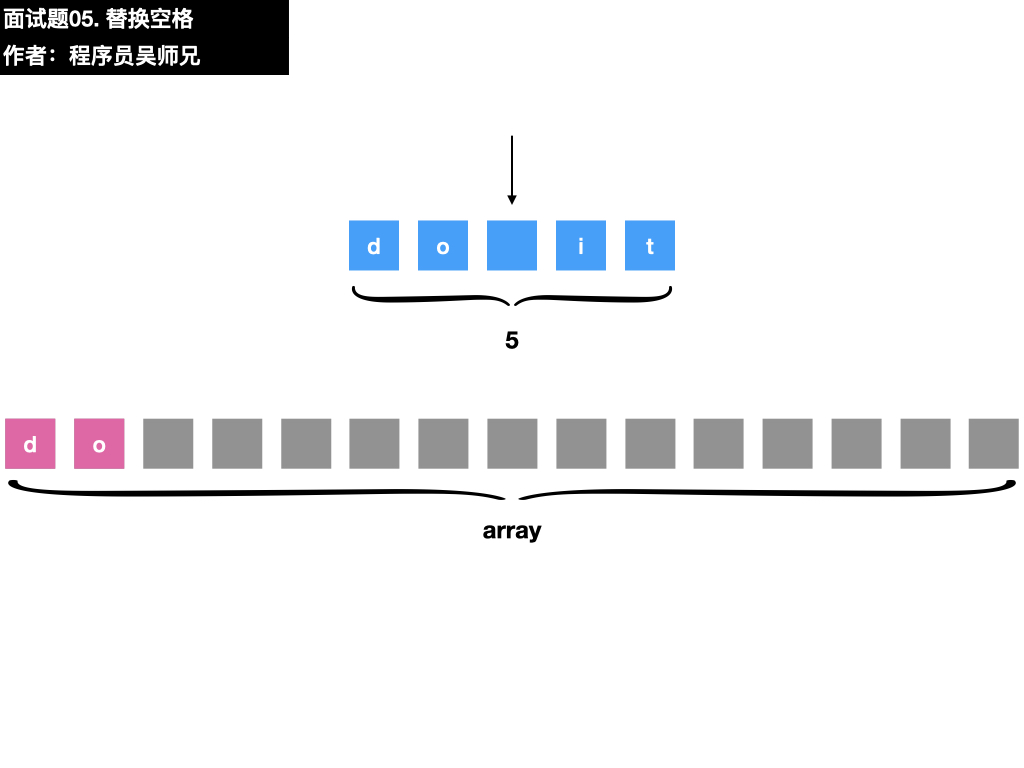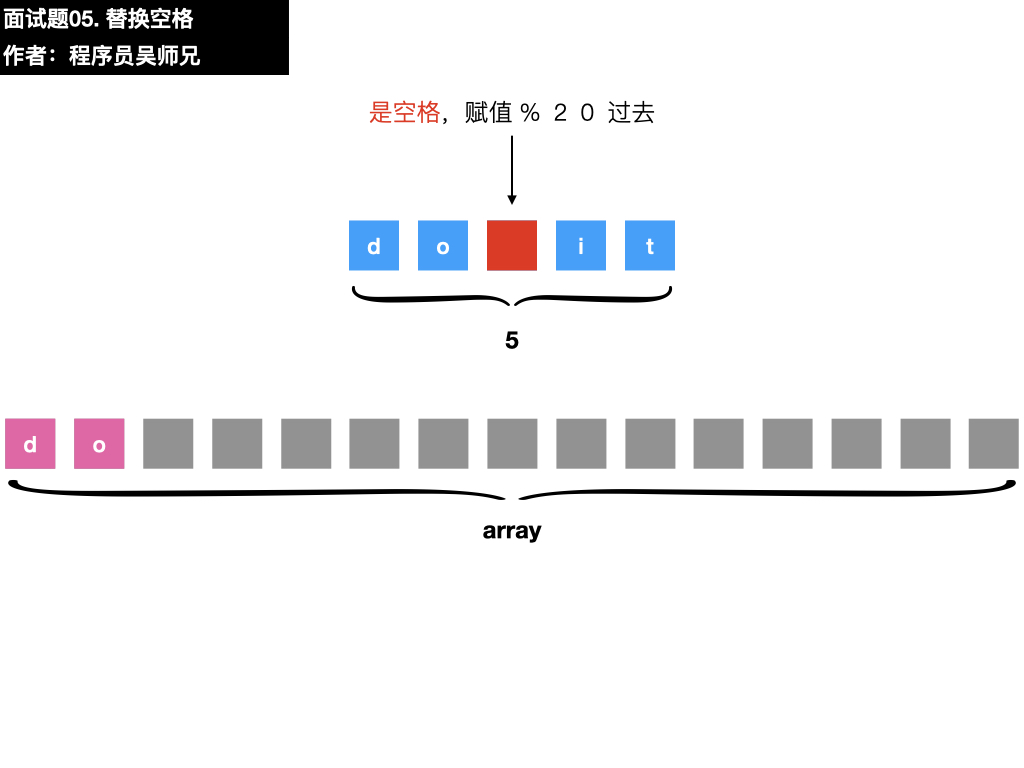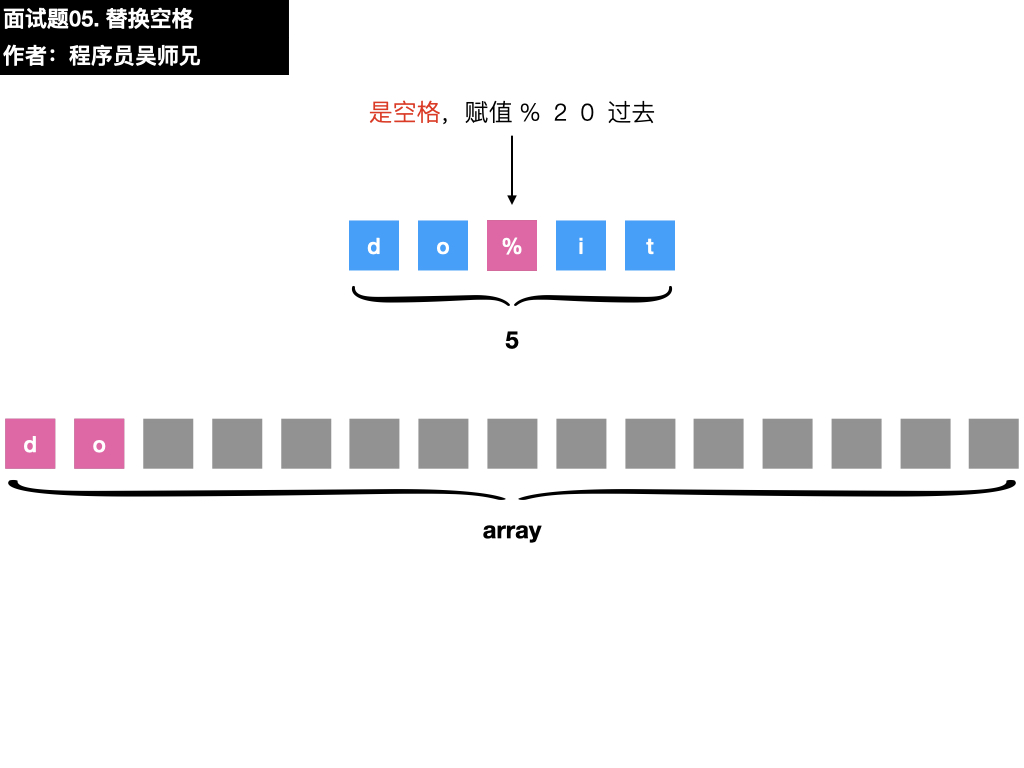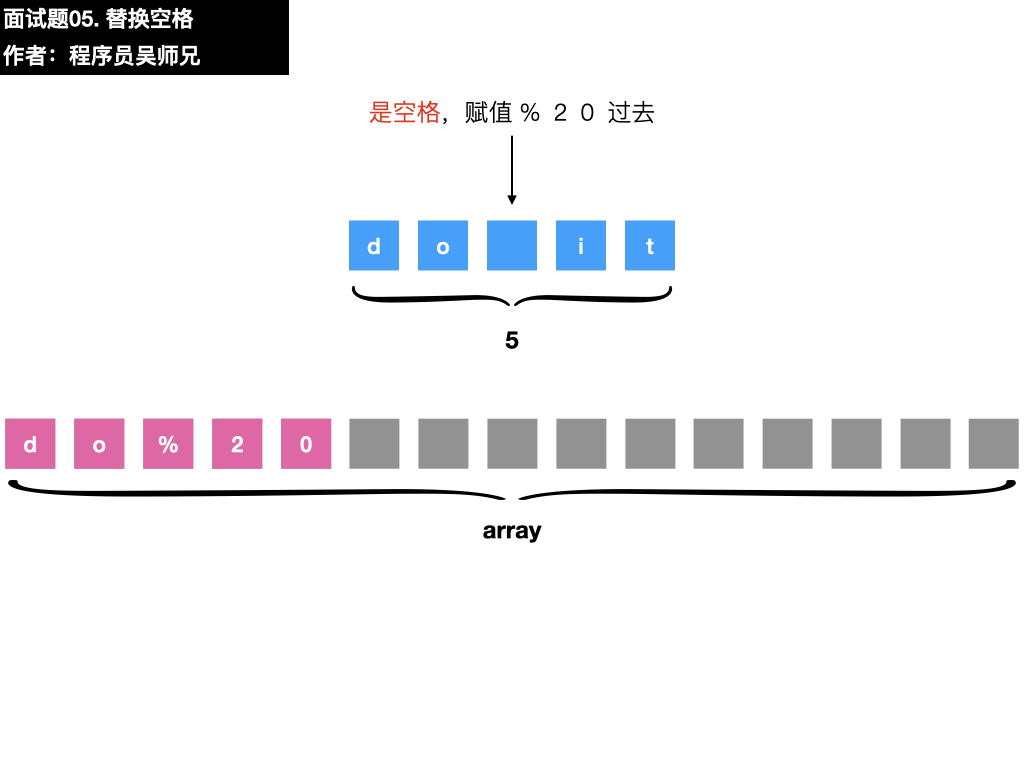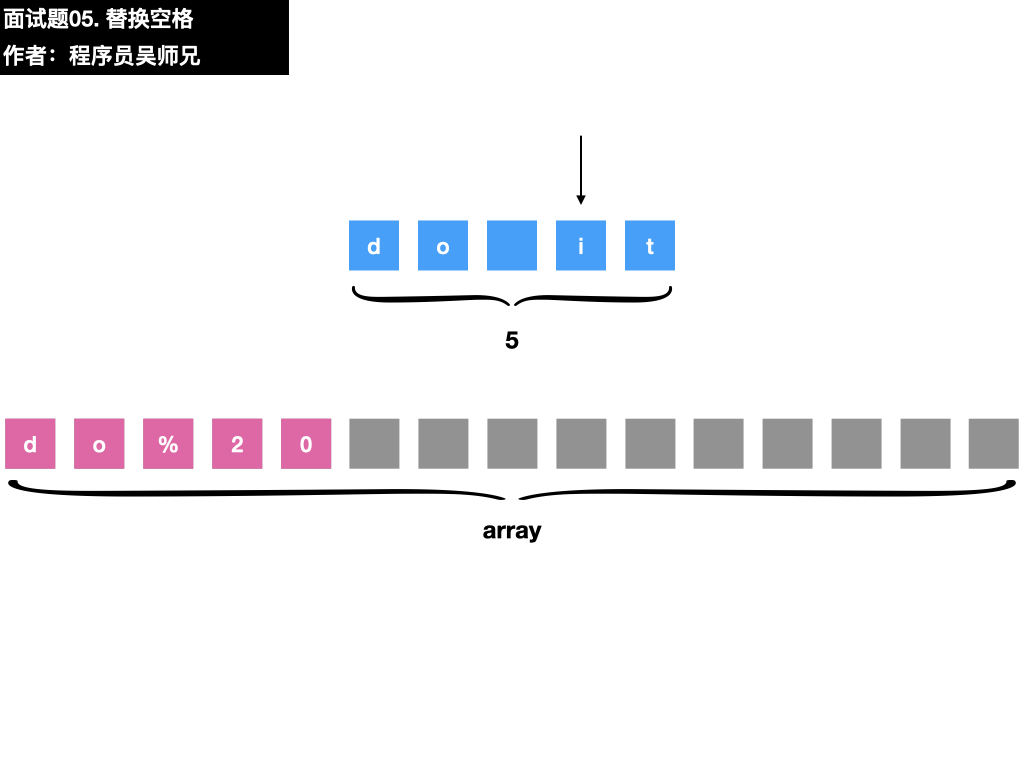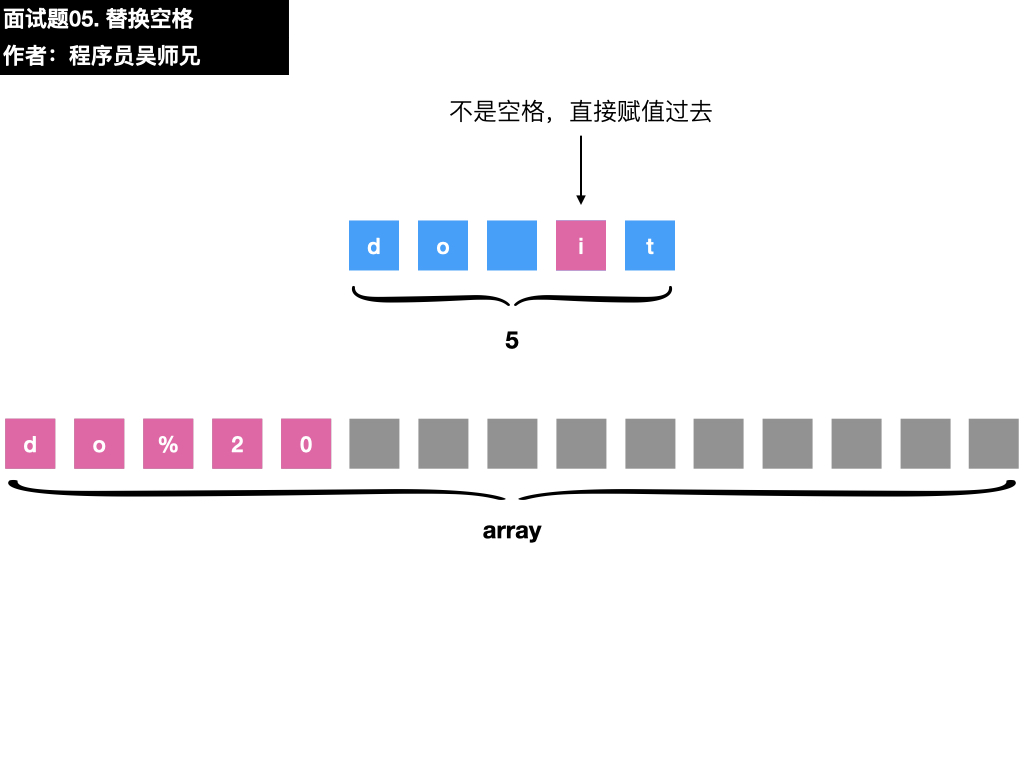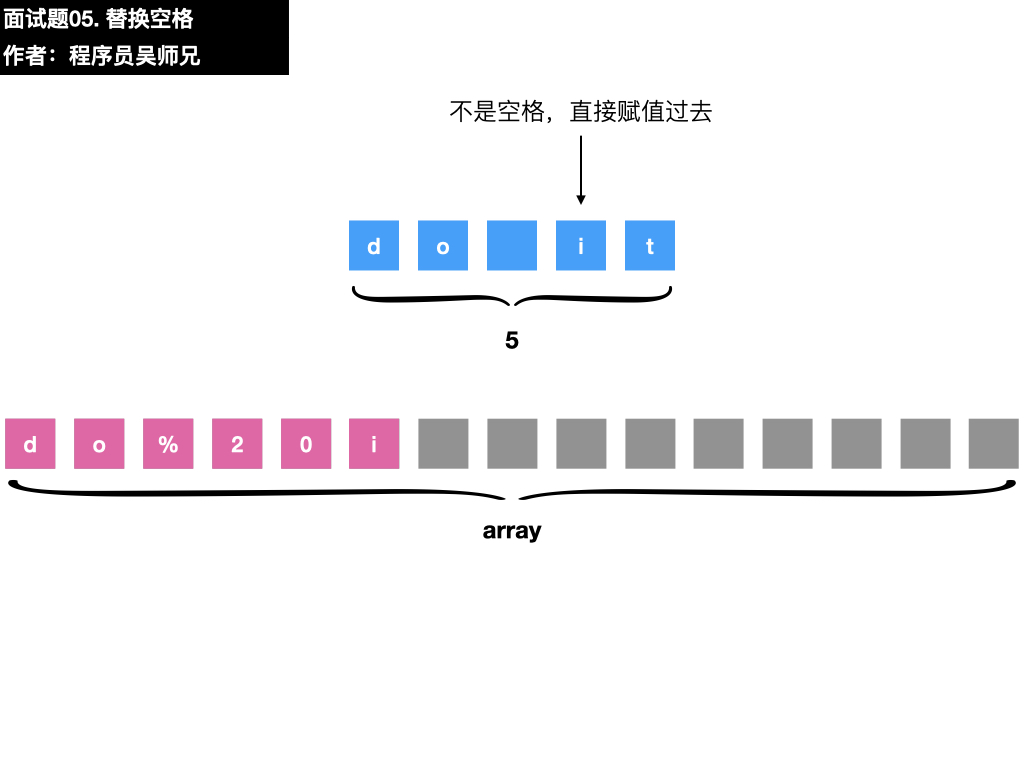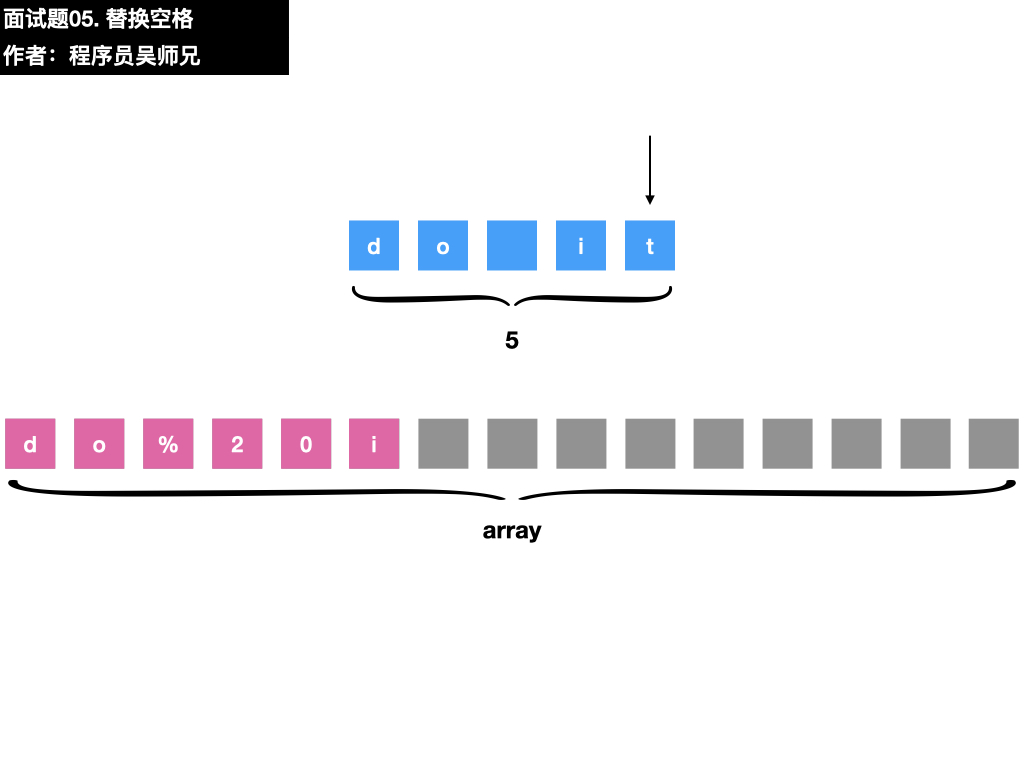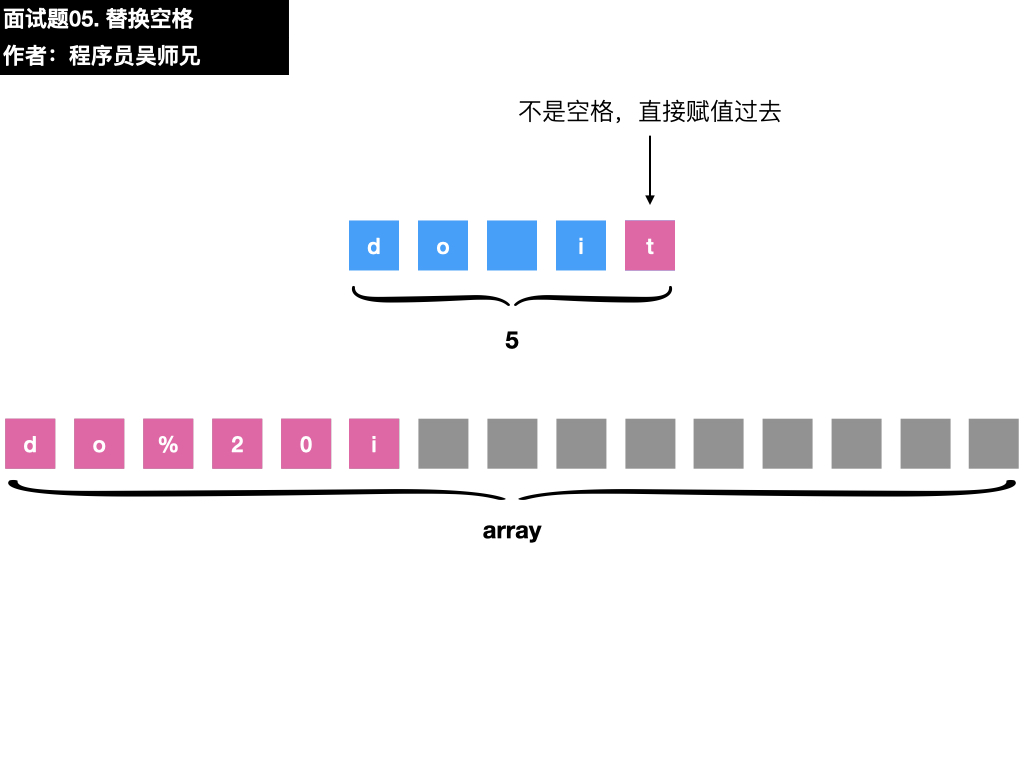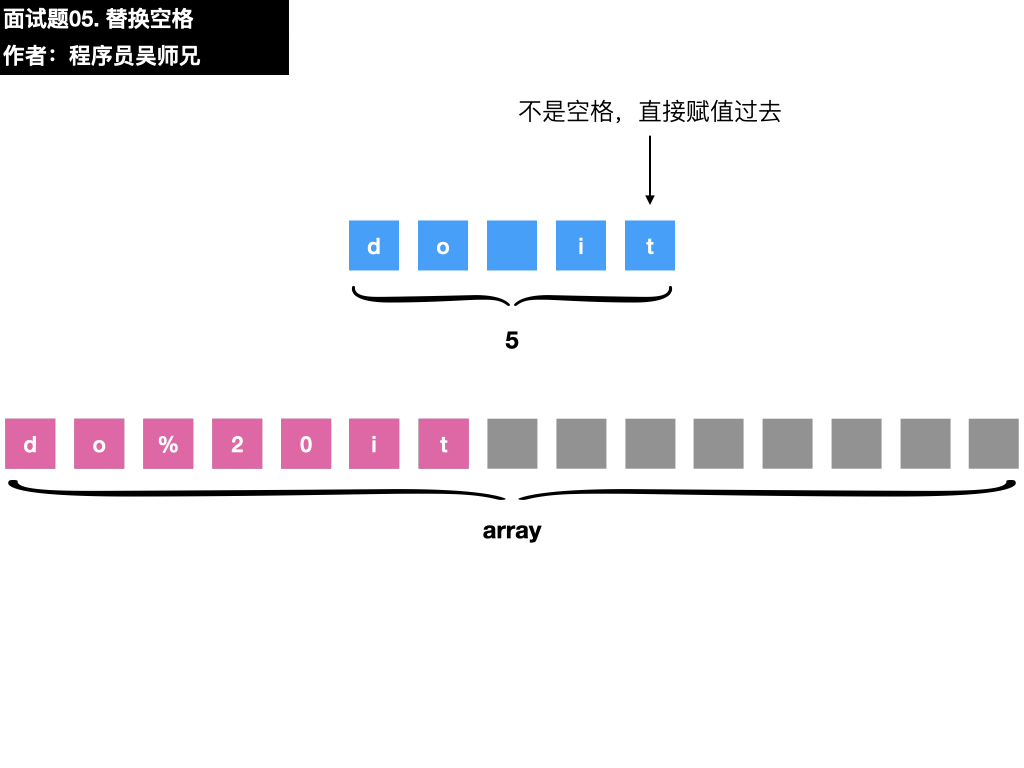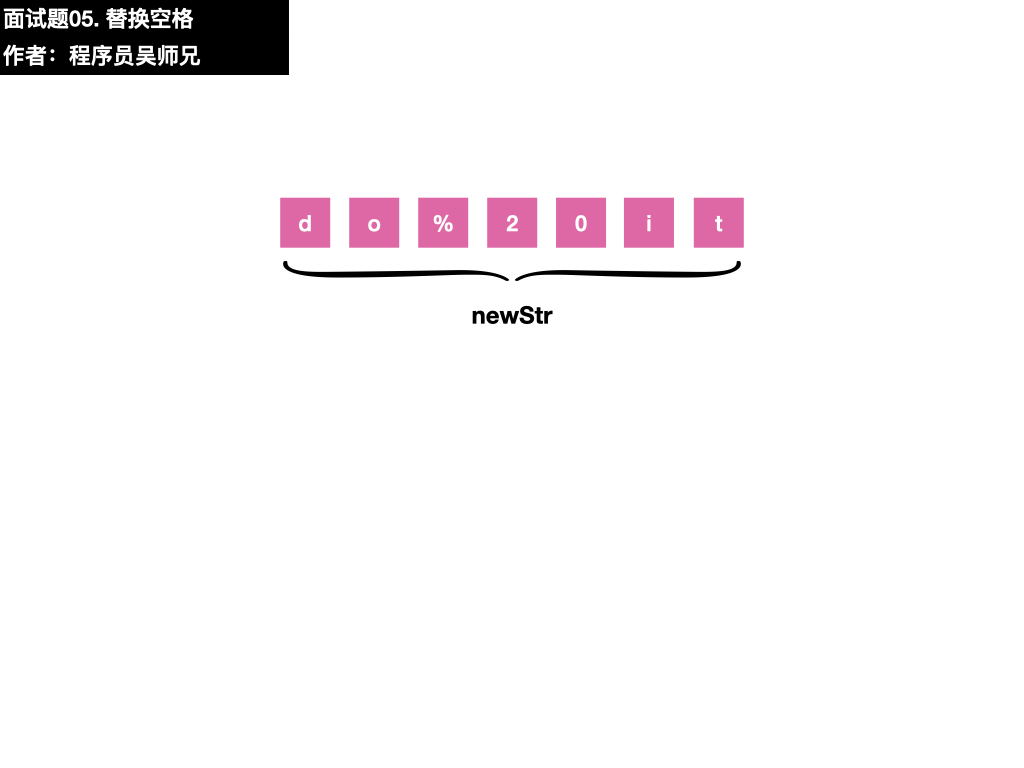### 五、参考代码

//来源：https://leetcode-cn.com/problems/ti-huan-kong-ge-lcof/solution/mian-shi-ti-05-ti-huan-kong-ge-by-leetcode-solutio/
class Solution {
public String replaceSpace(String s) {
//获取字符串 s 的长度 length
int length = s.length();
//创建字符数组 array，其长度为 length * 3
char[] array = new char[length * 3];
//初始化 size 为 0，size 表示替换后的字符串的长度
int size = 0;
//从左到右遍历字符串 s
for (int i = 0; i < length; i++) {
//获得 s 的当前字符 c
char c = s.charAt(i);
//如果字符 c 是空格，则令 array[size] = '%'，array[size + 1] = '2'，array[size + 2] = '0'，并将 size 的值加 3
if (c == ' ') {
array[size++] = '%';
array[size++] = '2';
array[size++] = '0';
} else {
array[size++] = c;
}
}
//遍历结束之后，size 的值等于替换后的字符串的长度，从 array 的前 size 个字符创建新字符串，并返回新字符串
String newStr = new String(array, 0, size);
return newStr;
}
}

• 字符串
• 数组